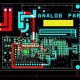# Best Tutorial on Conversion of Flip- Flip.

In this tutorial, we are going to learn about conversion of Flip- Flip.

In this tutorial we are going to learn about below topics:

1.  Characteristic table of desired flip-flop.
2. Fill the excitation values inputs of given flip-flop for each combination of present state and next state.

Tables of all flip flops are given here

• Simplified expressions for each excitation input. If needed, use K-maps for simplifying.
• Draw the circuit diagram of desired flip-flop according to the simplified expressions.

Conversion of some flip flops

1. SR flip-flop to D flip-flop conversion: given flip-flop is SR flip-flop and the desired flip-flop is D flip-flop. Characteristic table is given below.

SR flip-flop has two inputs S & R. So, excitation values of SR flip-flop for each combination of present state and next state values. Characteristic table of D flip-flop along with the excitation inputs of SR flip-flop.

Boolean functions for each input as below.

S=m2+d3S=m2+d3

R=m1+d0R=m1+d0

K-Maps for S & R are shown below.

Circuit diagram of D- flip flop

• D flip-flop to T flip-flop conversion

Characteristic table of T flip-flop.

D flip-flop has single input D. So, excitation values of D flip-flop for each combination of present state and next state values. Characteristic table of T flip-flop along with the excitation input of D flip-flop.

Boolean function of D as below.

D=T⊕Q(t)D=T⊕Q(t

Circuit diagram of T flip-flop

• JK flip-flop to T flip-flop conversion

Characteristic table of T flip-flop.

JK flip-flop has two inputs J & K. So excitation values of JK flip-flop for each combination of present state and next state values. Characteristic table of T flip-flop along with the excitation inputs of JK flipflop.

Boolean functions for each input as below.

J=m2+d1+d3J=m2+d1+d3

K=m3+d0+d2K=m3+d0+d2

K-Maps for J & K are shown below:-

.